# Credit Card Fraud - Imbalanced Data Set

Use Case: Credit Card Fraud Detection

Compare different common algorithms, develop and optimize a new 2 sequential/consecutive model algorithm to see if this can give better results



Author: Donald Stierman - Senior Data Scientist

Details: Imbalanced data can cause issues with most Machine Learning Algorithms and Neural Networks. To alleviate this, I choose to down-sample the training data to use as the input dataset. After creating the down-sampled dataset, I ran this through several different common model algorithms, including a new modeling technique I developed specifically for imbalanced data. I got this idea after reading about some highly effective Healthcare screening solutions currently in use. I.E. Breast Cancer detection in women (see comments: below). If a mammogram comes back positive, we already know that there will be a lot of false positives (benign tumors, scars, etc). Usually the doctor will follow up with a 2nd test, such as biopsy. This will screen out the false positives leaving mostly true positives (cancerous tissue). This same idea can possibly be applied to credit care fraud. We want to catch all true cases of fraud (fraud prevention), to be compliant with government regulations, and additionally not create a huge workload of false cases to be investigated (cost control).

Here are some different ways to explain the methodology used in the Healthcare use case:

*1st test (high specificity) -> 2nd test (high sensitivity) -> Only treat cancerous tissue

*TN/(TN + FP) is high ~ 1 -> TP/(TP + FN) is high ~ 1 -> Find all Positive cases

*catch all possible cases/remove healthy patients -> remove all false flags -> high confidence in Positive result/few missed positives

This same methodology can be applied to Credit Card Fraud detection

Link to code repo at Github:

https://github.com/donaldstierman/imbalanced_data

Models used:

    Logistic Regression
Random Forest
Customized 2 Step Gradient Boosted Decision Trees
Deep Neural Network
1D Convolutional Neural Network
AutoEncoder

Goal: For this example, I chose 2 metrics to optimize, ROC/AUC and best "macro avg recall". I chose these because in the health care example, it is better to catch all cancer patients, even if it means more tests are performed. To compare the results, first objective is to find the best overall model (lowest mislabelled predictions), second is to find the model that has a low number of false negatives (faudulent transactions that are missed) without having too many false positives (genuine transactions that are needlessly investigated)

    1) Compare the AUC to find the most robust model of the single step models. However, the value of this metric cannot be calculated directly on the 2 step model, so we need to use #2 below for final comparison
2) Maximize the Sensitivity (higher priority) or reduce the number of False Negatives (FN/TP ratio) and maximize the Specificity (lower priority) to control the number of tests performed in the 2nd step. I.E. catch all the fraudulent transaction even if there are false flags (false positives).

Results: The Customized 2 Step model has the best results overall, by only a slight margin.

                            AUC    Specificity/Sensitivity

Logistic Regression         .967    .95/.87
Random Forest               .977    .97/.89  **best AUC**
Customized 2 Step GB Trees  NA      .99/.93  **best overall**
Deep Neural Network         .973    .95/.92  **2nd best overall**
AutoEncoder                 .954    .88/.93



Final Results: ROC Curve comparision

In :
# Import Libraries
# try some of these ideas: https://www.tensorflow.org/tutorials/structured_data/imbalanced_data
import numpy as np
import pandas as pd

import os
import matplotlib as mpl
if os.environ.get('DISPLAY','') == '':
print('no display found. Using non-interactive Agg backend')
mpl.use('Agg')

import matplotlib.pyplot as plt
%matplotlib inline
import pandas_profiling as pp
import seaborn as sns

from sklearn.model_selection import train_test_split
from sklearn.linear_model import LogisticRegression
from sklearn.tree import DecisionTreeClassifier
from sklearn.ensemble import RandomForestClassifier
from sklearn import metrics
from sklearn.metrics import confusion_matrix
from sklearn.metrics import classification_report
from sklearn.metrics import roc_curve
from sklearn.metrics import roc_auc_score
from sklearn.metrics import precision_recall_curve
from sklearn.metrics import f1_score
from sklearn.metrics import auc
from sklearn.metrics import accuracy_score
from sklearn.metrics import precision_score
from sklearn.metrics import recall_score
from sklearn.metrics import cohen_kappa_score
from sklearn.utils.class_weight import compute_sample_weight
from sklearn.preprocessing import StandardScaler
from matplotlib import pyplot
import zipfile

import tensorflow as tf

no display found. Using non-interactive Agg backend


Always like to include a timer function to see where my code is running slow or taking most of the run time

In :
class MyTimer():
# usage:
#with MyTimer():
#    rf.fit(X_train, y_train)

def __init__(self):
self.start = time.time()
def __enter__(self):
return self
def __exit__(self, exc_type, exc_val, exc_tb):
end = time.time()
runtime = end - self.start
msg = 'The function took {time} seconds to complete'
print(msg.format(time=runtime))

In :
def CalcPct(df,title):
unique_elements, counts_elements = np.unique(df, return_counts=True)
calc_pct = round(counts_elements/(counts_elements+counts_elements) * 100,6)
print(title)
print(np.asarray((unique_elements, counts_elements)))
return calc_pct

In :
colab = os.environ.get('COLAB_GPU', '10')
if (int(colab) == 0):
drive.mount('/content/drive')
else:
print("")


Setup to run on Google Colab and Kaggle platforms

In :
# Check if Google Colab path exists
if os.path.exists("/content/drive/My Drive/MyDSNotebooks/Imbalanced_data/input/creditcardzip") :
# Change the current working Directory
os.chdir("/content/drive/My Drive/MyDSNotebooks/Imbalanced_data/input/creditcardzip")
# else check if Kaggle/local path exists
elif os.path.exists("../input/creditcardzip") :
# Change the current working Directory
os.chdir("../input/creditcardzip")
else:
print("Can't change the Current Working Directory")
print("Current Working Directory " , os.getcwd())

Current Working Directory  C:\DataScience\Repo\Imbalanced_data\CreditCardFraud\input\creditcardzip

In :
verbose=0
#off line data source for backup


Public Credit Card Dataset. This is financial data, and is considered to be sensitive so it is "encrypted" through the use of PCA to protect privacy. Only the Time and Dollar columns are intact after the "encryption"

Doing some initial data exploration

In :
# Check the data, make sure it loaded okay

   Time        V1        V2        V3        V4        V5        V6        V7  \
0   0.0 -1.359807 -0.072781  2.536347  1.378155 -0.338321  0.462388  0.239599
1   0.0  1.191857  0.266151  0.166480  0.448154  0.060018 -0.082361 -0.078803
2   1.0 -1.358354 -1.340163  1.773209  0.379780 -0.503198  1.800499  0.791461
3   1.0 -0.966272 -0.185226  1.792993 -0.863291 -0.010309  1.247203  0.237609
4   2.0 -1.158233  0.877737  1.548718  0.403034 -0.407193  0.095921  0.592941

V8        V9  ...       V21       V22       V23       V24       V25  \
0  0.098698  0.363787  ... -0.018307  0.277838 -0.110474  0.066928  0.128539
1  0.085102 -0.255425  ... -0.225775 -0.638672  0.101288 -0.339846  0.167170
2  0.247676 -1.514654  ...  0.247998  0.771679  0.909412 -0.689281 -0.327642
3  0.377436 -1.387024  ... -0.108300  0.005274 -0.190321 -1.175575  0.647376
4 -0.270533  0.817739  ... -0.009431  0.798278 -0.137458  0.141267 -0.206010

V26       V27       V28  Amount  Class
0 -0.189115  0.133558 -0.021053  149.62      0
1  0.125895 -0.008983  0.014724    2.69      0
2 -0.139097 -0.055353 -0.059752  378.66      0
3 -0.221929  0.062723  0.061458  123.50      0
4  0.502292  0.219422  0.215153   69.99      0

[5 rows x 31 columns]

In :
# Check the datatypes of the Data set
df.info()

<class 'pandas.core.frame.DataFrame'>
RangeIndex: 284807 entries, 0 to 284806
Data columns (total 31 columns):
#   Column  Non-Null Count   Dtype
---  ------  --------------   -----
0   Time    284807 non-null  float64
1   V1      284807 non-null  float64
2   V2      284807 non-null  float64
3   V3      284807 non-null  float64
4   V4      284807 non-null  float64
5   V5      284807 non-null  float64
6   V6      284807 non-null  float64
7   V7      284807 non-null  float64
8   V8      284807 non-null  float64
9   V9      284807 non-null  float64
10  V10     284807 non-null  float64
11  V11     284807 non-null  float64
12  V12     284807 non-null  float64
13  V13     284807 non-null  float64
14  V14     284807 non-null  float64
15  V15     284807 non-null  float64
16  V16     284807 non-null  float64
17  V17     284807 non-null  float64
18  V18     284807 non-null  float64
19  V19     284807 non-null  float64
20  V20     284807 non-null  float64
21  V21     284807 non-null  float64
22  V22     284807 non-null  float64
23  V23     284807 non-null  float64
24  V24     284807 non-null  float64
25  V25     284807 non-null  float64
26  V26     284807 non-null  float64
27  V27     284807 non-null  float64
28  V28     284807 non-null  float64
29  Amount  284807 non-null  float64
30  Class   284807 non-null  int64
dtypes: float64(30), int64(1)
memory usage: 67.4 MB

In :
# Check the Uniqueness
df.nunique()

Out:
Time      124592
V1        275663
V2        275663
V3        275663
V4        275663
V5        275663
V6        275663
V7        275663
V8        275663
V9        275663
V10       275663
V11       275663
V12       275663
V13       275663
V14       275663
V15       275663
V16       275663
V17       275663
V18       275663
V19       275663
V20       275663
V21       275663
V22       275663
V23       275663
V24       275663
V25       275663
V26       275663
V27       275663
V28       275663
Amount     32767
Class          2
dtype: int64
In :
# Check for missing data
df.isnull().sum()

Out:
Time      0
V1        0
V2        0
V3        0
V4        0
V5        0
V6        0
V7        0
V8        0
V9        0
V10       0
V11       0
V12       0
V13       0
V14       0
V15       0
V16       0
V17       0
V18       0
V19       0
V20       0
V21       0
V22       0
V23       0
V24       0
V25       0
V26       0
V27       0
V28       0
Amount    0
Class     0
dtype: int64
In :
# Check basic Statistics

df.describe(include ='all')

Out:
Time V1 V2 V3 V4 V5 V6 V7 V8 V9 ... V21 V22 V23 V24 V25 V26 V27 V28 Amount Class
count 284807.000000 2.848070e+05 2.848070e+05 2.848070e+05 2.848070e+05 2.848070e+05 2.848070e+05 2.848070e+05 2.848070e+05 2.848070e+05 ... 2.848070e+05 2.848070e+05 2.848070e+05 2.848070e+05 2.848070e+05 2.848070e+05 2.848070e+05 2.848070e+05 284807.000000 284807.000000
mean 94813.859575 1.165980e-15 3.416908e-16 -1.373150e-15 2.086869e-15 9.604066e-16 1.490107e-15 -5.556467e-16 1.177556e-16 -2.406455e-15 ... 1.656562e-16 -3.444850e-16 2.578648e-16 4.471968e-15 5.340915e-16 1.687098e-15 -3.666453e-16 -1.220404e-16 88.349619 0.001727
std 47488.145955 1.958696e+00 1.651309e+00 1.516255e+00 1.415869e+00 1.380247e+00 1.332271e+00 1.237094e+00 1.194353e+00 1.098632e+00 ... 7.345240e-01 7.257016e-01 6.244603e-01 6.056471e-01 5.212781e-01 4.822270e-01 4.036325e-01 3.300833e-01 250.120109 0.041527
min 0.000000 -5.640751e+01 -7.271573e+01 -4.832559e+01 -5.683171e+00 -1.137433e+02 -2.616051e+01 -4.355724e+01 -7.321672e+01 -1.343407e+01 ... -3.483038e+01 -1.093314e+01 -4.480774e+01 -2.836627e+00 -1.029540e+01 -2.604551e+00 -2.256568e+01 -1.543008e+01 0.000000 0.000000
25% 54201.500000 -9.203734e-01 -5.985499e-01 -8.903648e-01 -8.486401e-01 -6.915971e-01 -7.682956e-01 -5.540759e-01 -2.086297e-01 -6.430976e-01 ... -2.283949e-01 -5.423504e-01 -1.618463e-01 -3.545861e-01 -3.171451e-01 -3.269839e-01 -7.083953e-02 -5.295979e-02 5.600000 0.000000
50% 84692.000000 1.810880e-02 6.548556e-02 1.798463e-01 -1.984653e-02 -5.433583e-02 -2.741871e-01 4.010308e-02 2.235804e-02 -5.142873e-02 ... -2.945017e-02 6.781943e-03 -1.119293e-02 4.097606e-02 1.659350e-02 -5.213911e-02 1.342146e-03 1.124383e-02 22.000000 0.000000
75% 139320.500000 1.315642e+00 8.037239e-01 1.027196e+00 7.433413e-01 6.119264e-01 3.985649e-01 5.704361e-01 3.273459e-01 5.971390e-01 ... 1.863772e-01 5.285536e-01 1.476421e-01 4.395266e-01 3.507156e-01 2.409522e-01 9.104512e-02 7.827995e-02 77.165000 0.000000
max 172792.000000 2.454930e+00 2.205773e+01 9.382558e+00 1.687534e+01 3.480167e+01 7.330163e+01 1.205895e+02 2.000721e+01 1.559499e+01 ... 2.720284e+01 1.050309e+01 2.252841e+01 4.584549e+00 7.519589e+00 3.517346e+00 3.161220e+01 3.384781e+01 25691.160000 1.000000

8 rows × 31 columns

In :
# Check the Class Imbalance of the Data

df['Class'].value_counts()

Out:
0    284315
1       492
Name: Class, dtype: int64
In :
# Histograms of the features
# most of the data has a quasi-normal/gaussian distribution

df.hist(bins=20, figsize=(20,15))
plt.show()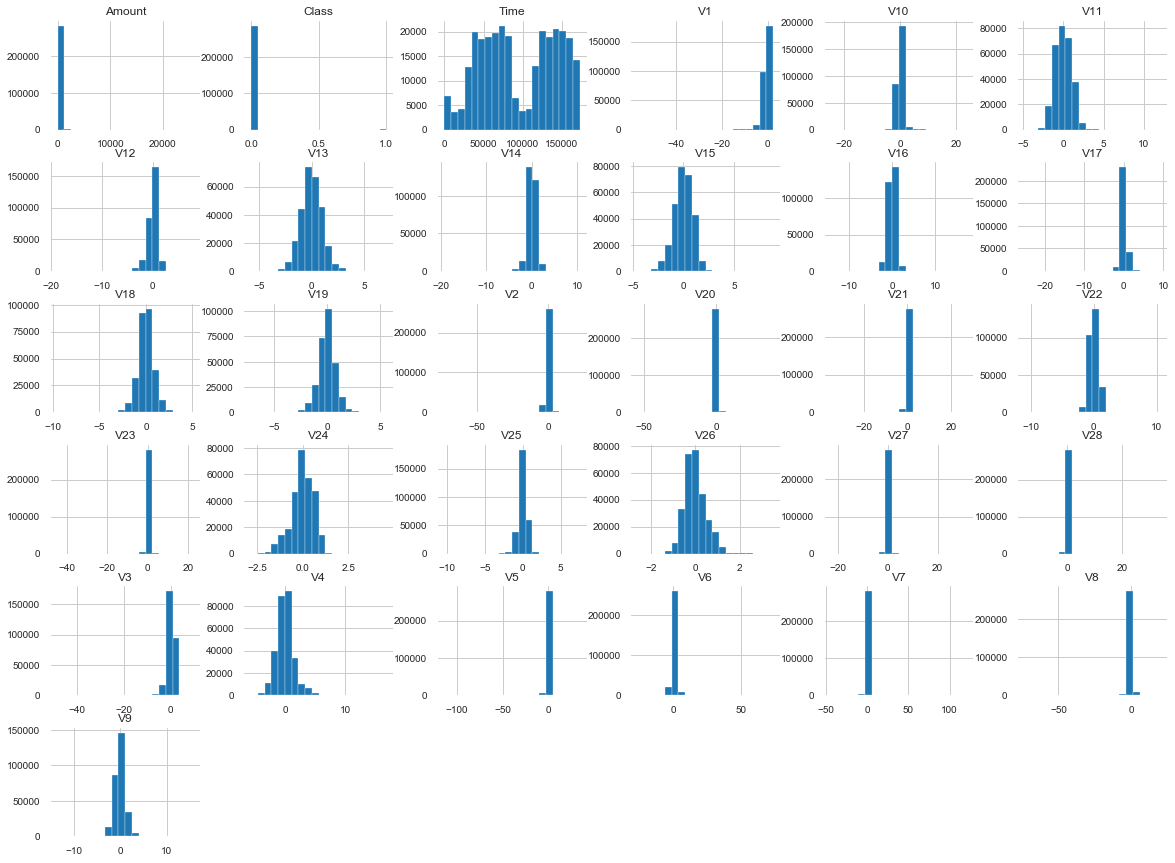Look at cross correlations between features. Most models will be fine with collinearity, but good to know this in any case. Most of my input is numerical, and my label is binary classification, so I can choose the Anova or Kendall's method. I will try the Kendall tau-b method first. This method will sort the 2 columns and compare if the X is always > or < Y. If so, the tau-b value will be 1.

Some key points to remember: Kendall’s Tau: Calculations based on concordant and discordant pairs. Insensitive to error. P values are more accurate with smaller sample sizes. Good resource can be found here: https://online.stat.psu.edu/stat509/node/158/

This image shows which method you should choose based on your dataset: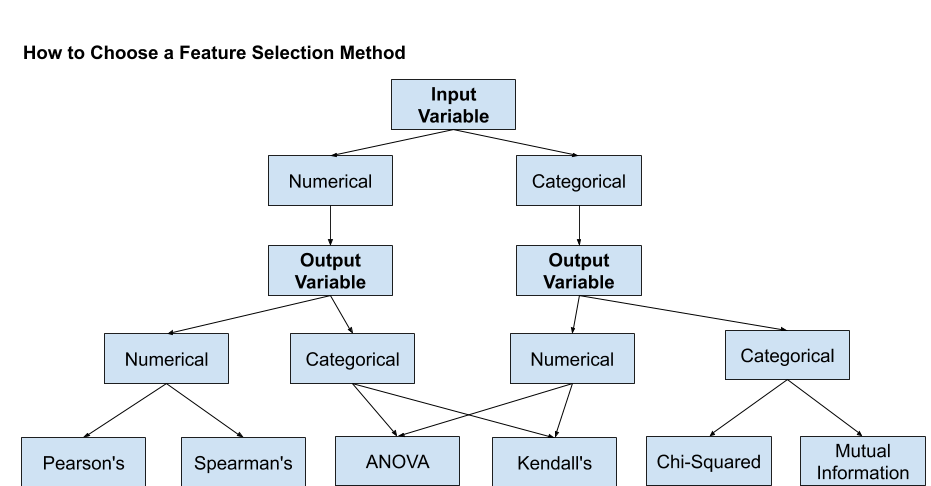In :
f = plt.figure(figsize=(19, 15))
plt.matshow(df.corr(method='kendall'), fignum=f.number) # pearson or spearman are also available
plt.xticks(range(df.shape), df.columns, fontsize=14, rotation=45)
plt.yticks(range(df.shape), df.columns, fontsize=14)
cb = plt.colorbar()
cb.ax.tick_params(labelsize=14)
plt.title('Correlation Matrix', fontsize=16)

Out:
Text(0.5, 1.05, 'Correlation Matrix')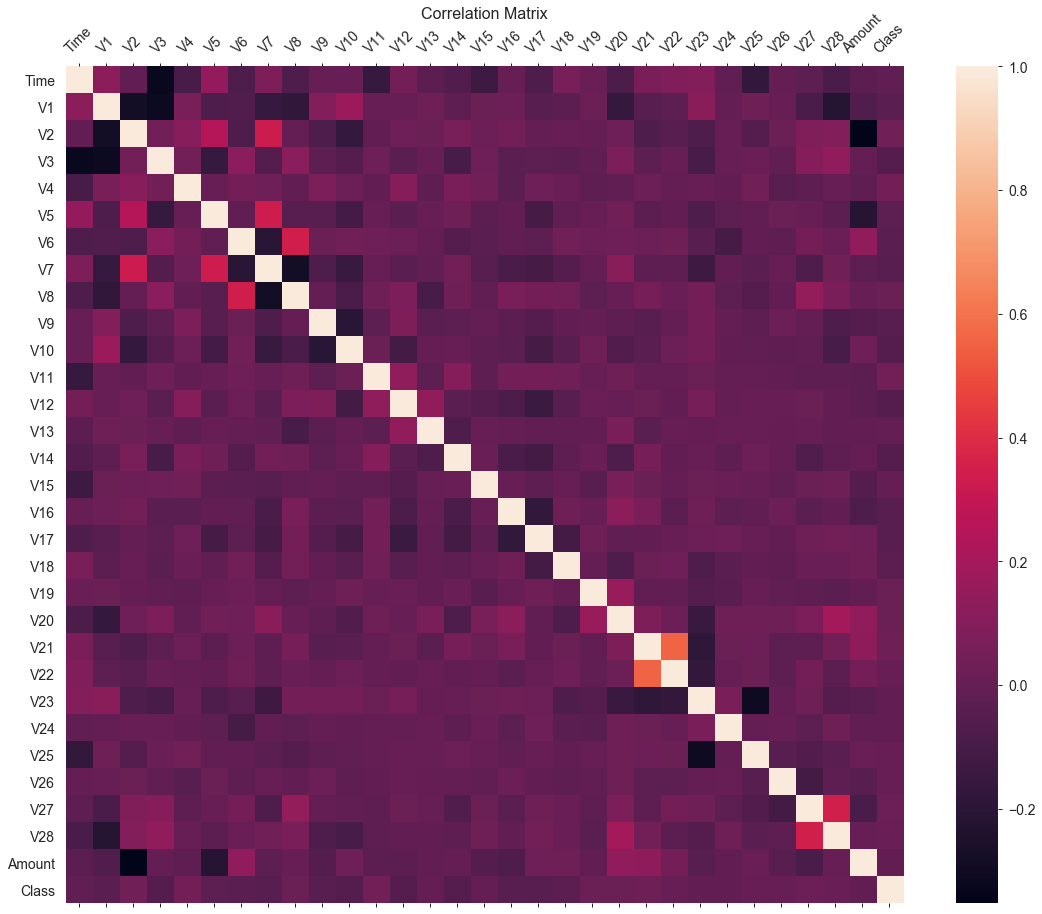V21 and V22 show the highest tau-b score, will investigate this relationship later

In :
#try some data cleansing, Amount has a few high values, so try using the log of that column instead.

temp_df = df.copy()
temp_df = temp_df.drop(['Time'], axis=1)
temp_df['Log_Amount'] = np.log(temp_df.pop('Amount')+0.001)
df = temp_df.copy()


Divide the dataset into features and labels and then into Train, Test and Validate datasets

In :
# divide full data into features and label
spl1 = 0.3
spl2 = 0.3
X = df.loc[:, df.columns != 'Class']
y = df.loc[:, df.columns == 'Class']
OrigPct = CalcPct(y,"Original")

strat = True
if (strat == True):
stratify=y['Class']
else:
stratify="None"
# create train, test and validate datasets

# first split original into Train and Test+Val
X_train, X_test1, y_train, y_test1 = train_test_split(X,y, test_size = spl1, random_state = None, shuffle=True, stratify=stratify)
# then split Test+Val into Test and Validate
# Validate will only be used in the 2 Model system (explained below)
X_test, X_val, y_test, y_val = train_test_split(X_test1,y_test1, test_size = spl2, random_state = None, shuffle=True)

Original
[[     0      1]
[284315    492]]

In :
# prepare data for model, need to do this normalization and clipping separately for X_train, X_test and X_val
# to avoid any contamination between Train and Test/Validate datasets

sc = StandardScaler()

scaled_features = StandardScaler().fit_transform(X_train.values)
X_train = pd.DataFrame(scaled_features, index=X_train.index, columns=X_train.columns)
scaled_features = StandardScaler().fit_transform(X_test.values)
X_test = pd.DataFrame(scaled_features, index=X_test.index, columns=X_test.columns)
scaled_features = StandardScaler().fit_transform(X_val.values)
X_val = pd.DataFrame(scaled_features, index=X_val.index, columns=X_val.columns)

# handle any extreme fliers, set to 5 or -5
X_train = np.clip(X_train, -5, 5)
X_test = np.clip(X_test, -5, 5)
X_val = np.clip(X_val, -5, 5)

In :
# Check basic Statistics after normalizing and clipping data

X_train.describe(include ='all')

Out:
V1 V2 V3 V4 V5 V6 V7 V8 V9 V10 ... V20 V21 V22 V23 V24 V25 V26 V27 V28 Log_Amount
count 199364.000000 199364.000000 199364.000000 199364.000000 199364.000000 199364.000000 199364.000000 199364.000000 199364.000000 199364.000000 ... 199364.000000 199364.000000 199364.000000 199364.000000 199364.000000 199364.000000 199364.000000 199364.000000 199364.000000 1.993640e+05
mean 0.009783 0.012029 0.005900 -0.001097 0.001759 -0.000254 0.002123 0.018824 -0.001106 -0.005705 ... 0.003860 -0.008809 0.000679 0.004067 -0.000252 0.000340 -0.000289 0.007916 -0.002568 1.971631e-16
std 0.917491 0.860434 0.947260 0.993028 0.892177 0.968165 0.832694 0.748990 0.988418 0.898173 ... 0.768171 0.713802 0.989699 0.699046 0.998613 0.990707 0.998391 0.799406 0.676540 1.000003e+00
min -5.000000 -5.000000 -5.000000 -3.955627 -5.000000 -5.000000 -5.000000 -5.000000 -5.000000 -5.000000 ... -5.000000 -5.000000 -5.000000 -5.000000 -4.666920 -5.000000 -5.000000 -5.000000 -5.000000 -4.870862e+00
25% -0.465785 -0.357587 -0.584669 -0.598174 -0.498882 -0.573427 -0.442805 -0.173540 -0.584856 -0.492151 ... -0.269671 -0.308746 -0.748016 -0.258805 -0.583077 -0.607519 -0.679977 -0.173353 -0.156136 -5.925446e-01
50% 0.011735 0.040645 0.119320 -0.014340 -0.040447 -0.205299 0.033661 0.019043 -0.047261 -0.084703 ... -0.078446 -0.040996 0.011442 -0.017245 0.067380 0.031434 -0.108753 0.003298 0.031359 7.510023e-02
75% 0.667124 0.482940 0.676204 0.524085 0.438765 0.297050 0.455866 0.273646 0.543689 0.418380 ... 0.173196 0.250633 0.728861 0.237051 0.725200 0.675233 0.501785 0.223727 0.225447 6.934260e-01
max 1.244339 5.000000 5.000000 5.000000 5.000000 5.000000 5.000000 5.000000 5.000000 5.000000 ... 5.000000 5.000000 5.000000 5.000000 5.000000 5.000000 5.000000 5.000000 5.000000 3.562634e+00

8 rows × 29 columns

In :
class_names=[0,1] # name  of classes 1=fraudulent transaction

y_val['Class'].value_counts()

TrainPct = CalcPct(y_train,"Train")
TestPct = CalcPct(y_test,"Train")
ValPct = CalcPct(y_val,"Train")
zeros, ones = np.bincount(y_train['Class'])

Train
[[     0      1]
[199020    344]]
Train
[[    0     1]
[59704   106]]
Train
[[    0     1]
[25591    42]]


Investigate the high tau-b value between V21 and V22

In :
# Form np arrays of labels and features for jointplot charts

train_labels = np.array(y_train).flatten()
bool_train_labels = train_labels != 0 # has an extra ,1 in the bool_train_labels.shape
val_labels = np.array(y_val)
test_labels = np.array(y_test)
train_features = np.array(X_train)
val_features = np.array(X_val)
test_features = np.array(X_test)

pos_df = pd.DataFrame(train_features[ bool_train_labels], columns = X.columns)
neg_df = pd.DataFrame(train_features[~bool_train_labels], columns = X.columns)
sns.jointplot(pos_df['V21'], pos_df['V22'],
kind='hex', xlim = (-5,5), ylim = (-5,5))
plt.suptitle("Positive distribution")
sns.jointplot(neg_df['V21'], neg_df['V22'],
kind='hex', xlim = (-5,5), ylim = (-5,5))
_ = plt.suptitle("Negative distribution")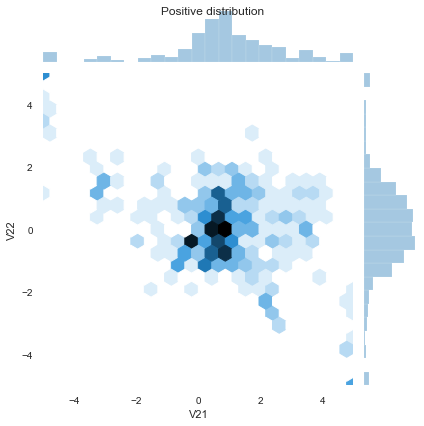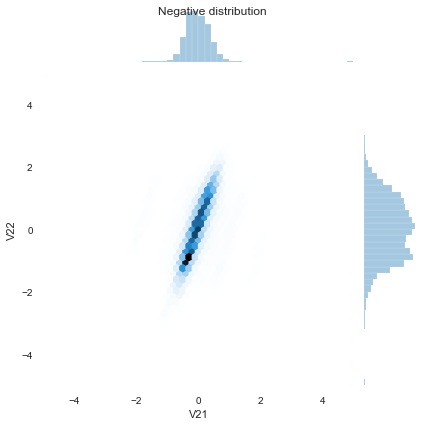V21 shows a slight one-sided tail, however Kendall's correlation test is good to use here as it is a non-parametric test and can handle non-gaussian distributions like this

For a imbalanced sampling strategy, I will be using undersampling in my project as i think this is the best approach for this type of data

In :
# find the number of minority (value=1) samples in our train set so we can down-sample our majority to it
yes = len(y_train[y_train['Class'] ==1])

# retrieve the indices of the minority and majority samples
yes_ind = y_train[y_train['Class'] == 1].index
no_ind = y_train[y_train['Class'] == 0].index

# random sample the majority indices based on the amount of
# minority samples
new_no_ind = np.random.choice(no_ind, yes, replace = False)

# merge the two indices together
undersample_ind = np.concatenate([new_no_ind, yes_ind])

# get undersampled dataframe from the merged indices of the train dataset
X_train = X_train.loc[undersample_ind]
y_train = y_train.loc[undersample_ind]

y_train = np.array(y_train).flatten()


Create some calculation and visualization functions to show the results

In :
def visualize(Actual, Pred, Algo):
#Confusion Matrix
cnf_matrix=metrics.confusion_matrix(Actual, Pred) #

#Visualize confusion matrix using heat map

fig, ax = plt.subplots()
tick_marks = np.arange(len(class_names))
plt.xticks(tick_marks, class_names)
plt.yticks(tick_marks, class_names)

# create heatmap
sns.heatmap(pd.DataFrame(cnf_matrix), annot=True, cmap="YlGnBu" ,fmt='g')
ax.xaxis.set_label_position("top")
plt.tight_layout()
plt.title('Confusion matrix: '+Algo, y=1.1)
plt.ylabel('Actual label')
plt.xlabel('Predicted label')
plt.show()

In :
def display_metrics(model_name, train_features, test_features, train_label, test_label, pred, algo):
model_probs = model_name.predict_proba(test_features)
n = model_name.predict_proba(test_features).shape-1
model_probs = model_probs[:, n]
try:
print(model_name.score(test_features, test_label))
print("Accuracy score (training): {0:.3f}".format(model_name.score(train_features, train_label)))
print("Accuracy score (validation): {0:.3f}".format(model_name.score(test_features, test_label)))
except Exception as e:
print("error")
try:
print(pd.Series(model_name.feature_importances_, index=train_features.columns[:]).nlargest(10).plot(kind='barh'))
except Exception as e:
print("error")
print("Confusion Matrix:")
tn, fp, fn, tp = confusion_matrix(test_label, pred).ravel()
total = tn+ fp+ fn+ tp
print("false positive pct:",(fp/total)*100)
print("tn", " fp", " fn", " tp")
print(tn, fp, fn, tp)
print(confusion_matrix(test_label, pred))
print("Classification Report")
print(classification_report(test_label, pred))
print("Specificity =", tn/(tn+fp))
print("Sensitivity =", tp/(tp+fn))
y=np.reshape(test_label.to_numpy(), -1)
fpr, tpr, thresholds = metrics.roc_curve(y, model_probs, pos_label=1)
cm_results.append([algo, tn, fp, fn, tp])
cr_results.append([algo, classification_report(test_label, pred)])
roc.append([algo, fpr, tpr, thresholds])
# AUC score should be (Sensitivity+Specificity)/2
print(algo + ':TEST | AUC Score: ' + str( round(metrics.auc(fpr, tpr),3 )))
return tn, fp, fn, tp

In :
def auc_roc_metrics(model, test_features, test_labels, algo): # model object, features, actual labels, name of algorithm
# useful for imbalanced data
ns_probs = [0 for _ in range(len(test_labels))]
# predict probabilities
model_probs = model.predict_proba(test_features)
# keep probabilities for the positive outcome only
n = model.predict_proba(test_features).shape-1
model_probs = model_probs[:, n]
model_auc = auc_roc_metrics_plots(model_probs, ns_probs, test_labels, algo)
return model_auc

In :
def auc_roc_metrics_plots(model_probs, ns_probs, test_labels, algo):

# calculate scores
ns_auc = roc_auc_score(test_labels, ns_probs) # no skill
model_auc = round(roc_auc_score(test_labels, model_probs), 3)

# summarize scores
print('%10s : ROC AUC=%.3f' % ('No Skill',ns_auc))
print('%10s : ROC AUC=%.3f' % (algo,model_auc))
# calculate roc curves
ns_fpr, ns_tpr, _ = roc_curve(test_labels, ns_probs)
# NameError: name 'ns_probs' is not defined
model_fpr, model_tpr, _ = roc_curve(test_labels, model_probs)
# plot the roc curve for the model
pyplot.plot(ns_fpr, ns_tpr, linestyle='--', label='No Skill')
pyplot.plot(model_fpr, model_tpr, marker='.', label='%s (area = %0.2f)' % (algo, model_auc))
# axis labels
pyplot.xlabel('False Positive Rate')
pyplot.ylabel('True Positive Rate')
# show the legend
pyplot.legend()
# show the plot
pyplot.show()
return model_auc

In :
# Define our custom loss function
def focal_loss(y_true, y_pred):
gamma = 2.0
alpha = 0.25
pt_1 = tf.where(tf.equal(y_true, 1), y_pred, tf.ones_like(y_pred))
pt_0 = tf.where(tf.equal(y_true, 0), y_pred, tf.zeros_like(y_pred))
return -K.sum(alpha * K.pow(1. - pt_1, gamma) * K.log(pt_1))-K.sum((1-alpha) * K.pow( pt_0, gamma) * K.log(1. - pt_0))

In :
def prediction_cutoff(model, test_features, cutoff):
model.predict_proba(test_features)
# to get the probability in each class,
# for example, first column is probability of y=0 and second column is probability of y=1.

# the probability of being y=1
prob1=model.predict_proba(test_features)[:,1]
predicted=[1 if i > cutoff else 0 for i in prob1]
return predicted

In :
metrics_results = {}
roc = []
cm_results = []
cr_results = []

In :
X_train.hist(bins=20, figsize=(20,15))
plt.show()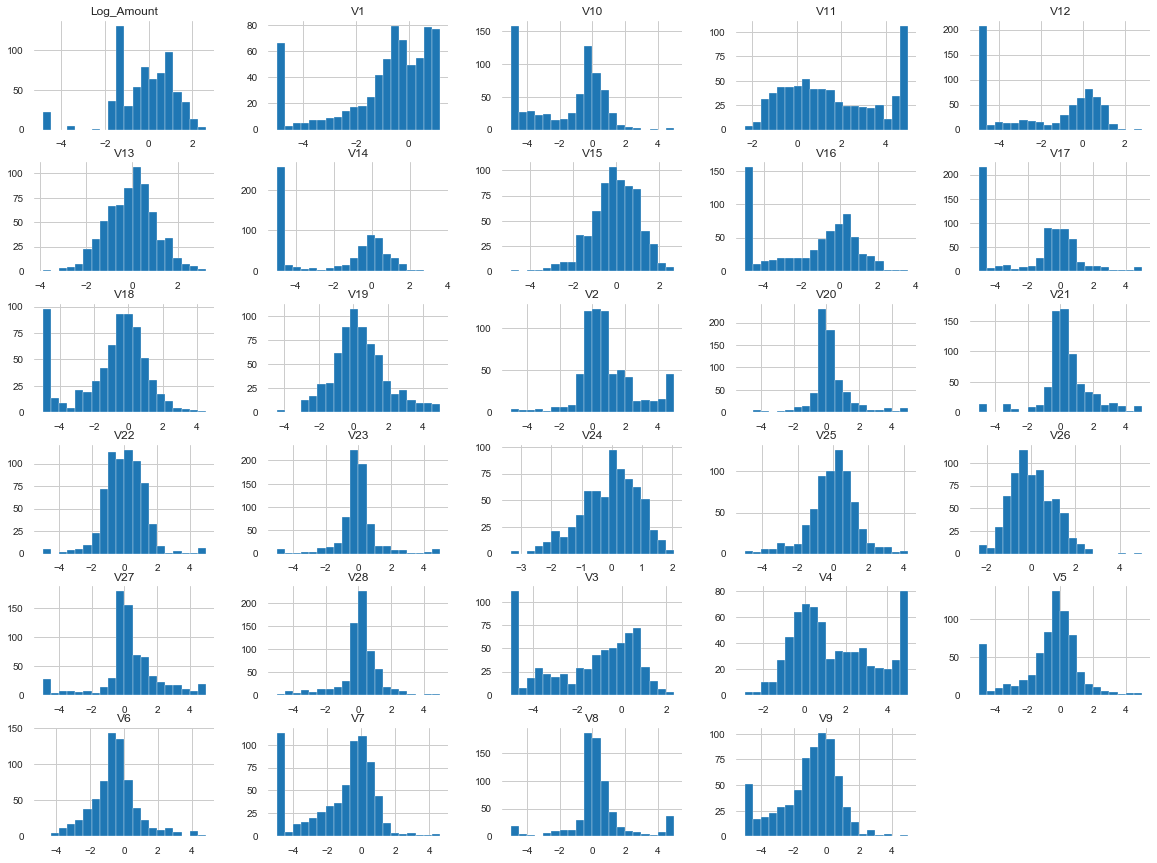run Logistic Regression model first

In :
lr = LogisticRegression()
#lr = LogisticRegression(solver='lbfgs')

lr.fit(X_train, y_train)
#lr_Pred = lr.predict(X_test)
# or
lr_Pred = prediction_cutoff(lr, X_test, 0.5) # 0.5 is the default cutoff for a logistic regression test


Show the results of this model

In :
print(metrics.accuracy_score(y_test, lr_Pred))
tn, fp, fn, tp = display_metrics(lr, X_train, X_test, y_train, y_test, lr_Pred, 'LR')
visualize(y_test, lr_Pred, 'LR') # actual labels vs predicted labels
lr_auc = auc_roc_metrics(lr, X_test, y_test, 'LR')
metrics_results['lr'] = lr_auc

0.9546564119712423
0.9546564119712423
Accuracy score (training): 0.955
Accuracy score (validation): 0.955
error
Confusion Matrix:
false positive pct: 4.520983113191774
tn  fp  fn  tp
57000 2704 8 98
[[57000  2704]
[    8    98]]
Classification Report
precision    recall  f1-score   support

0       1.00      0.95      0.98     59704
1       0.03      0.92      0.07       106

accuracy                           0.95     59810
macro avg       0.52      0.94      0.52     59810
weighted avg       1.00      0.95      0.98     59810

Specificity = 0.9547099021841082
Sensitivity = 0.9245283018867925
LR:TEST | AUC Score: 0.973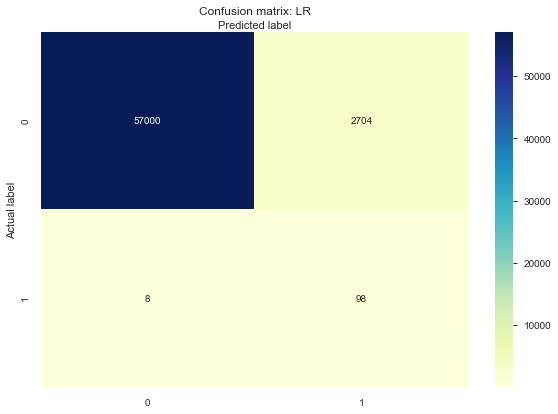No Skill : ROC AUC=0.500
LR : ROC AUC=0.973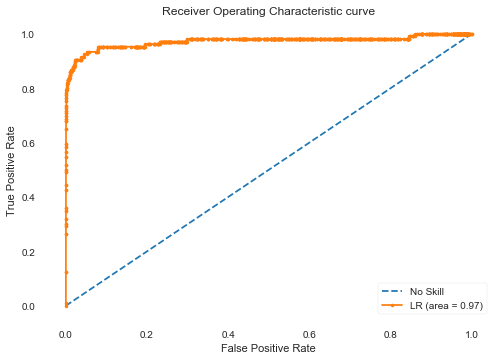In :
# useful for unbalanced data, maybe include later in metrics summary for all models

lr_precision, lr_recall, _ = precision_recall_curve(y_test, lr_Pred)
lr_f1, lr_auc = f1_score(y_test, lr_Pred), auc(lr_recall, lr_precision)
# summarize scores
print('Logistic: f1=%.3f auc=%.3f' % (lr_f1, lr_auc))
# plot the precision-recall curves
no_skill = len(y_test[y_test==1]) / len(y_test)
pyplot.plot([0, 1], [no_skill, no_skill], linestyle='--', label='No Skill')
pyplot.plot(lr_recall, lr_precision, marker='.', label='Logistic')
# axis labels
pyplot.xlabel('Recall')
pyplot.ylabel('Precision')
# show the legend
pyplot.legend()
# show the plot
pyplot.show()

Logistic: f1=0.067 auc=0.480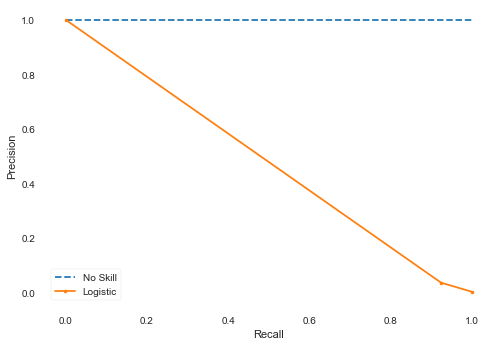Next try the Random Forest model

In :
rf = RandomForestClassifier(n_estimators = 1000)

rf.fit(X_train, y_train, sample_weight=np.where(y_train == 1,1.0,1.0).flatten())

rf_Pred=rf.predict(X_test)


Show the results of this model

In :
#print(metrics.accuracy_score(y_test, y_pred))
print(classification_report(y_test, rf_Pred))
tn, fp, fn, tp = display_metrics(rf, X_train, X_test, y_train, y_test, rf_Pred, 'RF')
visualize(y_test, rf_Pred, 'RF')
rf_auc = auc_roc_metrics(rf, X_test, y_test, 'RF')
metrics_results['rf'] = rf_auc

              precision    recall  f1-score   support

0       1.00      0.97      0.98     59704
1       0.05      0.91      0.09       106

accuracy                           0.97     59810
macro avg       0.52      0.94      0.54     59810
weighted avg       1.00      0.97      0.98     59810

0.9676809898010366
Accuracy score (training): 1.000
Accuracy score (validation): 0.968
AxesSubplot(0.125,0.125;0.775x0.755)
Confusion Matrix:
false positive pct: 3.215181407791339
tn  fp  fn  tp
57781 1923 10 96
[[57781  1923]
[   10    96]]
Classification Report
precision    recall  f1-score   support

0       1.00      0.97      0.98     59704
1       0.05      0.91      0.09       106

accuracy                           0.97     59810
macro avg       0.52      0.94      0.54     59810
weighted avg       1.00      0.97      0.98     59810

Specificity = 0.9677911027736835
Sensitivity = 0.9056603773584906
RF:TEST | AUC Score: 0.978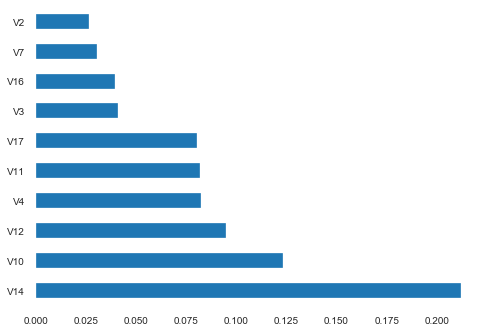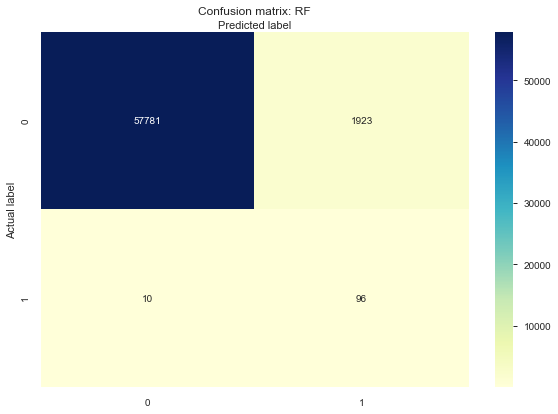No Skill : ROC AUC=0.500
RF : ROC AUC=0.978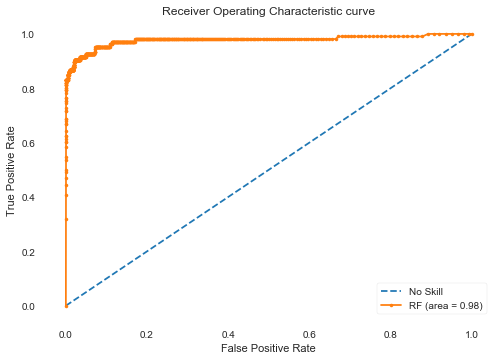There is some variability in the results from run to run, due to random sampling and imbalanced data. This time, LogisticRegression has better prediction capability, the RandomForestClassifier test has a lot more mistakes in the False Positive category, and even a few more mistakes in the False Negative category.

Now lets try a GradientBoosting Algorithm

In :
#setup model parameters, change some of the defaults based on benchmarking
max_depth=3, random_state=None, subsample = 0.5, criterion='mse',
min_samples_split = 10, min_samples_leaf = 10)

#default fit model
#gb_clf.fit(X_train, y_train)

#since a false negative is much more likely than a false positive, we should weight them accordingly
gb_clf.fit( X_train, y_train, sample_weight=np.where(y_train == 1,1.0,1.0) ) #  fn = 12 and fp = 1057
# no weights gives worse false positive counts
#gb_clf.fit( X_train, y_train) # fn = 8 and fp = 2639

#use model to predict validation dataset
predictions = gb_clf.predict(X_test)


Display the results

In :
tn, fp, fn, tp = display_metrics(gb_clf, X_train, X_test, y_train, y_test, predictions, 'GB')
visualize(y_test, predictions, 'GB')
gb_auc = auc_roc_metrics(gb_clf, X_test, y_test, 'GB')
metrics_results['gb'] = gb_auc

0.980036783146631
Accuracy score (training): 0.949
Accuracy score (validation): 0.980
AxesSubplot(0.125,0.125;0.775x0.755)
Confusion Matrix:
false positive pct: 1.9779301120214012
tn  fp  fn  tp
58521 1183 11 95
[[58521  1183]
[   11    95]]
Classification Report
precision    recall  f1-score   support

0       1.00      0.98      0.99     59704
1       0.07      0.90      0.14       106

accuracy                           0.98     59810
macro avg       0.54      0.94      0.56     59810
weighted avg       1.00      0.98      0.99     59810

Specificity = 0.9801855822055474
Sensitivity = 0.8962264150943396
GB:TEST | AUC Score: 0.973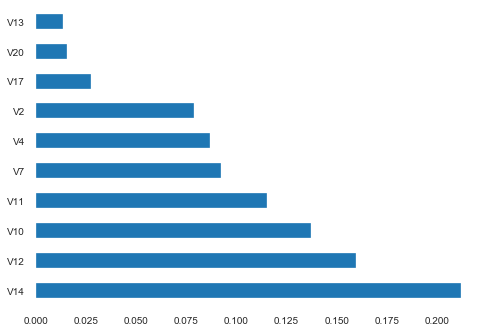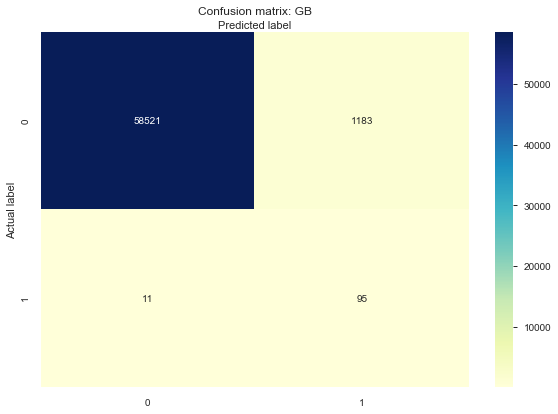No Skill : ROC AUC=0.500
GB : ROC AUC=0.973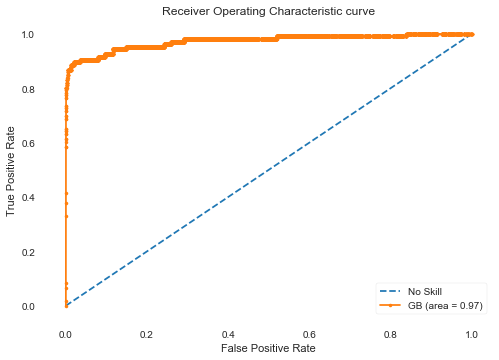After tweaking the parameters, i can get a decent result from GradientBoostingClassifier. Changing the weights has a very large influence on the number of errors (FN and FP). Since this data is mostly 0 values, decreasing the weight of a true value vs a false value will decrease the FN, doing the opposite will decrease the FP. For one example run: the sample_weight=np.where(y_train == 1,0.37,1.0) gives 13 FN and 795 FP. sample_weight=np.where(y_train == 1,0.1,1.0) gives 17 FN and 217 FP

My next idea is to run 2 consecutive models consecutively. 1st model should have low false negatives to catch (almost) all the actual positives, even if the number of false positives is high. Then only take these records with a predicted 1 value (should only be a few thousand), as the input for the next model. 2nd test should have low false positives to weed out the actual negatives. Will use the Validate dataset on the 2 models created from the Train and Test datasets

Here are some details on the new model:

Current: Full Dataset -> Train -> Build M1(Train) -> Run M1(Test) -> Filter(Predicted 1's from Test) -> Build M2 -> run M2(Filtered Test) Test

To Do:
Full Dataset -> Train -> Build M1(Train) -> Run M1(Test) -> Filter(Predicted 1's from Test) -> Build M2 -> run M1 and M2(Validate) Test Validate

Can also try the inverse, but think that option will have less chance of success.

1st step

build the 1st model to be used later on the validate dataset

In :
#setup model parameters, change some of the defaults based on benchmarking
max_depth=3, random_state=None, subsample = 1.0, criterion='mse',
min_samples_split = 10, min_samples_leaf = 10)

#default fit model
#gb_clf1.fit(X_train, y_train)

#since a false negative is much more likely than a false positive, we should weight them accordingly.
#IE Finding a true one is more important, also more rare
gb_clf1.fit( X_train, y_train, sample_weight=np.where(y_train == 1,3.6,1.4) ) # was 5.0

#use model to predict validation dataset
predictions = gb_clf1.predict(X_test)

In :
algo = 'GB1 Train **'
tn1, fp1, fn1, tp1 = display_metrics(gb_clf1, X_train, X_test, y_train, y_test, predictions, algo)
visualize(y_test, predictions, algo)
gb1_auc = auc_roc_metrics(gb_clf1, X_test, y_test, algo)
metrics_results['gb1_train'] = gb1_auc

0.9380036783146631
Accuracy score (training): 0.967
Accuracy score (validation): 0.938
AxesSubplot(0.125,0.125;0.775x0.755)
Confusion Matrix:
false positive pct: 6.18792844006019
tn  fp  fn  tp
56003 3701 7 99
[[56003  3701]
[    7    99]]
Classification Report
precision    recall  f1-score   support

0       1.00      0.94      0.97     59704
1       0.03      0.93      0.05       106

accuracy                           0.94     59810
macro avg       0.51      0.94      0.51     59810
weighted avg       1.00      0.94      0.97     59810

Specificity = 0.9380108535441511
Sensitivity = 0.9339622641509434
GB1 Train **:TEST | AUC Score: 0.978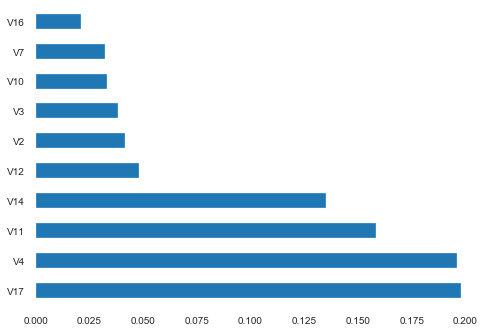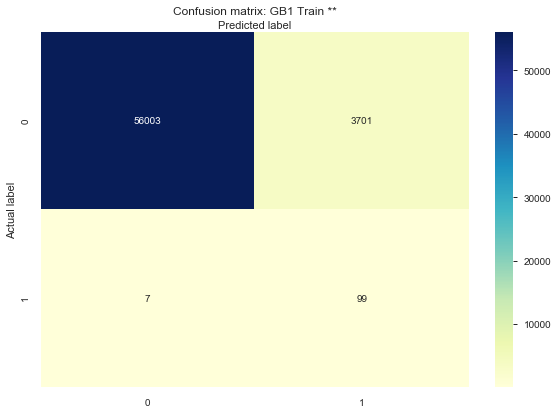No Skill : ROC AUC=0.500
GB1 Train ** : ROC AUC=0.978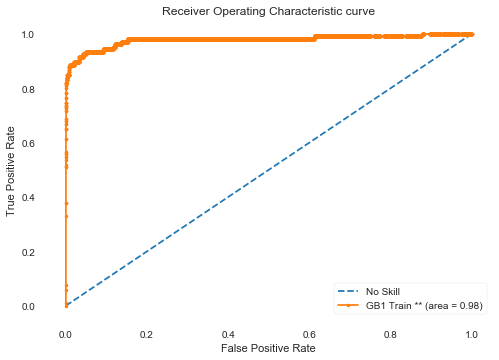2nd step takes all the Predicted Positives (the misclassified FP from upper right (~ 14000) plus the TP (since we won't use the actual value until the validation step)) and reprocesses these using a different model. The other 2 squares (Predicted 0's) are not included in the 2nd model, since we already have a low False negative result, so the initial predicted 0s don't change. Will need to add those back into the final results at the end.

Add 1st model prediction column to X_test for filtering

In :
X_test['Prediction'] = predictions


select rows with prediction of 1

In :
yes_ind = X_test[X_test['Prediction'] == 1].index


Create 2nd train dataset from 1st dataset where the prediction was 1

In :
X2_test = X_test.loc[yes_ind]
y2_test = y_test.loc[yes_ind]


clean up the X_test dataset for future modeling, means remove the Prediction column

In :
X_test = X_test.drop(['Prediction'], axis=1)
X2_test = X2_test.drop(['Prediction'], axis=1)


Look at the prediction values from the first model (preda_1) for the rows with a predicted label of 0

In :
proba = gb_clf1.predict_proba(X2_test)
pred = gb_clf1.predict(X2_test)
df = pd.DataFrame(data=proba[:,0], columns=["preda_1"])
df.hist(bins=20, figsize=(10,5))
plt.show()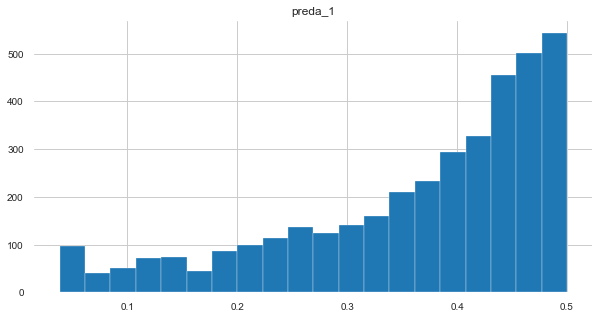Then we look at the ROC curve

In :
algo = 'PredictedPositives'
test_labels = y2_test
ns_probs = [0 for _ in range(len(test_labels))]
auc_roc_metrics_plots(proba[:,1], ns_probs, test_labels, algo)

  No Skill : ROC AUC=0.500
PredictedPositives : ROC AUC=0.961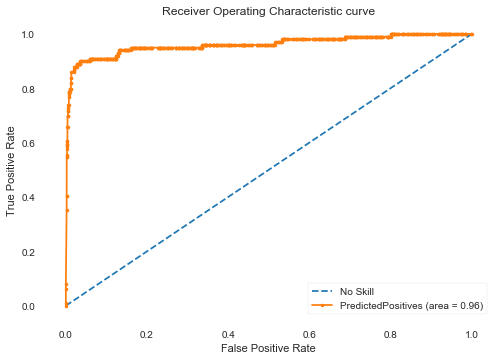Out:
0.961

Next we build the 2nd model to be used model later on the validate dataset and look at the output

In :
#setup model parameters, change some of the defaults based on benchmarking
max_depth=3, random_state=None, subsample = 1.0, criterion='mse',
min_samples_split = 10, min_samples_leaf = 10)

#default fit model
#gb_clf2.fit(X_train, y_train)

#since a false negative is much more likely than a false positive, we should weight them accordingly.
#IE Finding a true one is more important
# note that the weights in the 2nd model are the inverse of the weights in the 1st model
gb_clf2.fit( X_train, y_train, sample_weight=np.where(y_train == 1,3.6,1.4) ) # was 0.1 but should be > 1 to work correctly

#use model to predict validation dataset
predictions = gb_clf2.predict(X2_test)

algo = 'GB2 Train **'
tn, fp, fn, tp = display_metrics(gb_clf2, X_train, X2_test, y_train, y2_test, predictions, algo)

visualize(y2_test, predictions, algo)

gb2_auc = auc_roc_metrics(gb_clf2, X2_test, y2_test, algo)
metrics_results['gb2_train'] = gb2_auc

print("2 Step Final Confusion Matrix:")
print(tn+tn1, fp)
print(fn+fn1, tp)

fig, ax = plt.subplots()
tick_marks = np.arange(len(class_names))
plt.xticks(tick_marks, class_names)
plt.yticks(tick_marks, class_names)

#create heatmap with combined data from both models
sns.heatmap(pd.DataFrame([[tn+tn1,fp],[fn+fn1,tp]]), annot=True, cmap="YlGnBu" ,fmt='g')
ax.xaxis.set_label_position("top")
plt.tight_layout()
plt.title('2 Step Final Confusion matrix (Test)', y=1.1)
plt.ylabel('Actual label')
plt.xlabel('Predicted label')

0.29157894736842105
Accuracy score (training): 0.974
Accuracy score (validation): 0.292
AxesSubplot(0.125,0.125;0.775x0.755)
Confusion Matrix:
false positive pct: 70.84210526315789
tn  fp  fn  tp
1009 2692 0 99
[[1009 2692]
[   0   99]]
Classification Report
precision    recall  f1-score   support

0       1.00      0.27      0.43      3701
1       0.04      1.00      0.07        99

accuracy                           0.29      3800
macro avg       0.52      0.64      0.25      3800
weighted avg       0.97      0.29      0.42      3800

Specificity = 0.27262901918400434
Sensitivity = 1.0
GB2 Train **:TEST | AUC Score: 0.961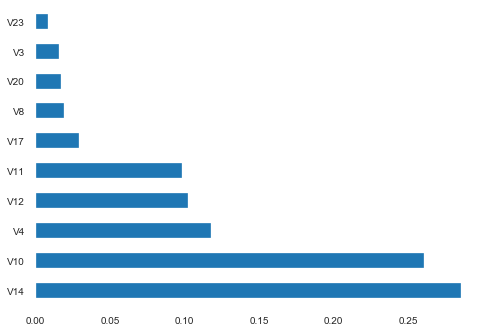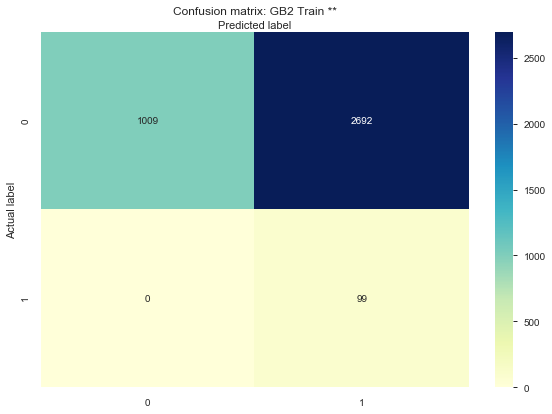No Skill : ROC AUC=0.500
GB2 Train ** : ROC AUC=0.961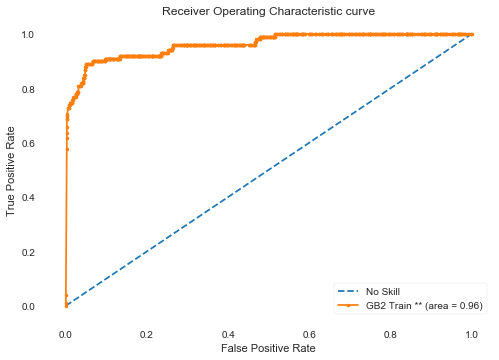2 Step Final Confusion Matrix:
57012 2692
7 99

Out:
Text(0.5, 352.48, 'Predicted label')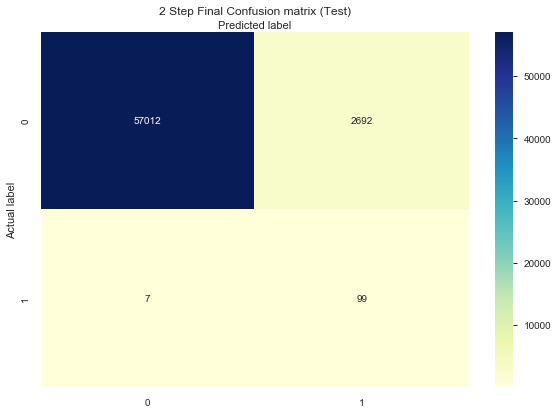Now that we have built the 2 models from the test dataset, run the untouched validate dataset through both of them to get an unbiased result to compare against

In :
# run the validate dataset through the first model
algo = '2-Step'
predictions1 = gb_clf1.predict(X_val)
predictions_proba1 = gb_clf1.predict_proba(X_val)
X1_val_final = X_val.copy()
X1_val_final=X1_val_final.join(y_val)
X1_val_final['Proba_1'] = predictions_proba1[:,1]
#X1_val_final
#X_val = X_val.sort_index(axis = 0)

In :
# adding this
# use both models to predict final validation dataset
algo = 'GB1 Validate **'
tn1, fp1, fn1, tp1 = display_metrics(gb_clf1, X_test, X_val, y_test, y_val, predictions1, algo)
visualize(y_val, predictions1, algo)
gb1_auc = auc_roc_metrics(gb_clf1, X_val, y_val, algo)
metrics_results['gb1_validate'] = gb1_auc

0.9395310732259197
Accuracy score (training): 0.938
Accuracy score (validation): 0.940
AxesSubplot(0.125,0.125;0.775x0.755)
Confusion Matrix:
false positive pct: 6.035189014161432
tn  fp  fn  tp
24044 1547 3 39
[[24044  1547]
[    3    39]]
Classification Report
precision    recall  f1-score   support

0       1.00      0.94      0.97     25591
1       0.02      0.93      0.05        42

accuracy                           0.94     25633
macro avg       0.51      0.93      0.51     25633
weighted avg       1.00      0.94      0.97     25633

Specificity = 0.9395490602164823
Sensitivity = 0.9285714285714286
GB1 Validate **:TEST | AUC Score: 0.986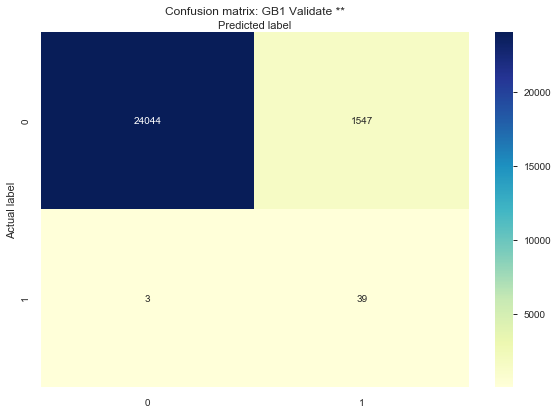No Skill : ROC AUC=0.500
GB1 Validate ** : ROC AUC=0.986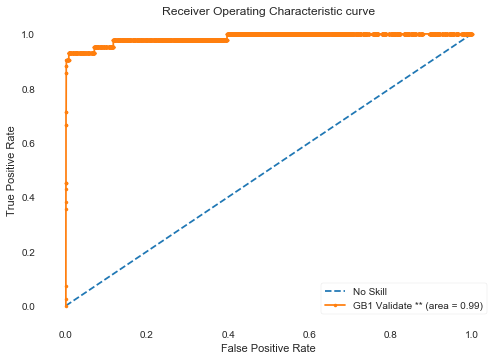In :
X_val['Prediction'] = predictions1

yes_ind = X_val[X_val['Prediction'] == 1].index

X2_val = X_val.loc[yes_ind]
y2_val = y_val.loc[yes_ind]
X2_val = X2_val.drop(['Prediction'], axis=1)
# run the validate dataset through the second model
predictions2 = gb_clf2.predict(X2_val)

X2_val_final = X2_val.copy()
X2_val_final.join(y2_val)
predictions_proba2 = gb_clf2.predict_proba(X2_val)
# validate the join!!
X2_val_final['Proba_2'] = predictions_proba2[:,1]
X2_val_final

cols_to_use = X2_val_final.columns.difference(X1_val_final.columns)
X_val_final = X1_val_final.join(X2_val_final[cols_to_use], how='left', lsuffix='_1', rsuffix='_2')
# rowwise action (axis=1)
X_val_final.loc[X_val_final['Proba_2'].isnull(),'Proba_2'] = X_val_final['Proba_1']
#X_val_final['Proba_2'].fillna(df['Proba_1'])
#X_val_final.query("Proba_1 != Proba_2")

#remove this column for use later
X_val = X_val.drop(['Prediction'], axis=1)

In :
algo = 'GB2 Validate **'
tn, fp, fn, tp = display_metrics(gb_clf2, X_train, X2_val, y_train, y2_val, predictions2, algo)
visualize(y2_val, predictions2, algo)
gb2_auc = auc_roc_metrics(gb_clf2, X2_val, y2_val, algo)
metrics_results['gb2_validate'] = gb2_auc

print("2 Step Final Confusion Matrix:")
print(tn+tn1, fp)
print(fn+fn1, tp)

fig, ax = plt.subplots()
tick_marks = np.arange(len(class_names))
plt.xticks(tick_marks, class_names)
plt.yticks(tick_marks, class_names)

#create heatmap with combined data from both models
sns.heatmap(pd.DataFrame([[tn+tn1,fp],[fn+fn1,tp]]), annot=True, cmap="YlGnBu" ,fmt='g')
ax.xaxis.set_label_position("top")
plt.tight_layout()
plt.title('2 Step Final Confusion matrix (Validate)', y=1.1)
plt.ylabel('Actual label')
plt.xlabel('Predicted label')

algo = '2-Step'
Specificity = (tn+tn1)/(tn+tn1+fp)
Sensitivity = tp/(tp+fn+fn1)

print("Specificity =", Specificity)
print("Sensitivity =", Sensitivity)

print('2 Step Algorithm' + ':TEST | AUC Score: ' + str( round( (Specificity+Sensitivity)/2,3 )))

cm_results.append([algo, (tn+tn1), fp, (fn+fn1), tp])
# HERE
#two_step_auc = auc_roc_metrics(gb_clf, X_test, y_test, '2-Step')

0.2931904161412358
Accuracy score (training): 0.974
Accuracy score (validation): 0.293
AxesSubplot(0.125,0.125;0.775x0.755)
Confusion Matrix:
false positive pct: 70.68095838587641
tn  fp  fn  tp
426 1121 0 39
[[ 426 1121]
[   0   39]]
Classification Report
precision    recall  f1-score   support

0       1.00      0.28      0.43      1547
1       0.03      1.00      0.07        39

accuracy                           0.29      1586
macro avg       0.52      0.64      0.25      1586
weighted avg       0.98      0.29      0.42      1586

Specificity = 0.27537168713639304
Sensitivity = 1.0
GB2 Validate **:TEST | AUC Score: 0.992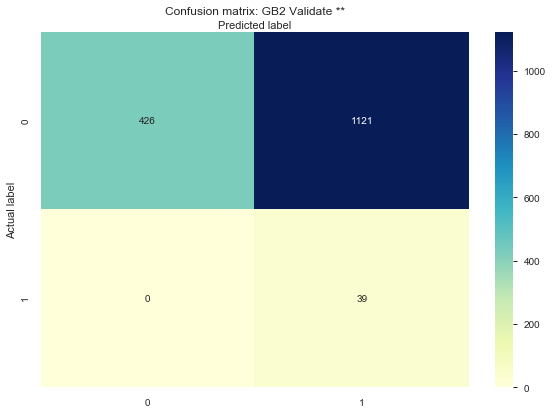No Skill : ROC AUC=0.500
GB2 Validate ** : ROC AUC=0.992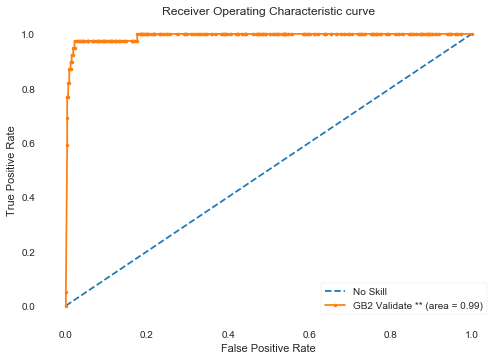2 Step Final Confusion Matrix:
24470 1121
3 39
Specificity = 0.9561955374936502
Sensitivity = 0.9285714285714286
2 Step Algorithm:TEST | AUC Score: 0.942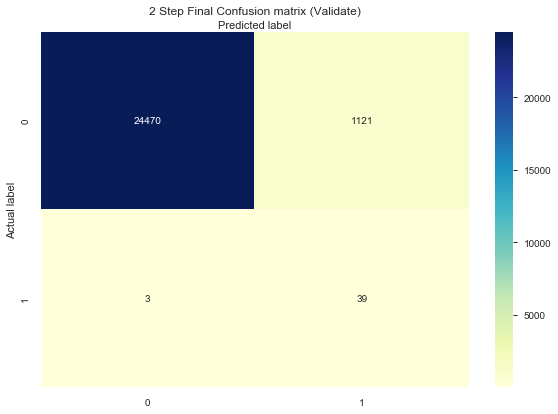In :
# try to combine the 2 models into one AUC score, however not sure that the proba values from 2 different models can be combined

test_labels = X_val_final['Class']
ns_probs = [0 for _ in range(len(test_labels))]
model_probs = X_val_final['Proba_2']
model_pred=[1 if i > 0.50 else 0 for i in model_probs]

two_step_auc = auc_roc_metrics_plots(model_probs, ns_probs, test_labels, algo)

metrics_results['2-step'] = two_step_auc

cr_results.append([algo, classification_report(test_labels, model_pred)])

  No Skill : ROC AUC=0.500
2-Step : ROC AUC=0.986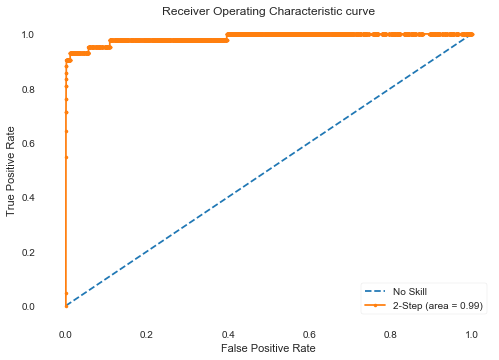In :
y=np.reshape(test_labels.to_numpy(), -1)
fpr, tpr, thresholds = metrics.roc_curve(y, model_probs, pos_label=1)
roc.append([algo, fpr, tpr, thresholds])


The 2 step process has the highest sensitivity (and specificity) between the models. The 2 step process also improves the overall model prediction of positives by a large amount (FP/TP ratio from above 10x to below 2x). I don't think we could get this high of precision and recall together with a single model. The best I could do with a single model was 10x FP/TP ratio.

Next will try a few Neural Networks

In :
import keras
from keras.models import Sequential
from keras.layers import Dense
from keras.layers import Dropout
from keras.layers import LSTM
from keras.layers import Embedding
#from keras.callbacks import ModelCheckpoint, TensorBoard
from keras import regularizers
from keras import backend as K
from keras.layers import Conv1D
from keras.layers import BatchNormalization
from keras.layers import MaxPool1D
from keras.layers import Flatten
from keras.backend import sigmoid
from keras.utils.generic_utils import get_custom_objects
from keras.layers import Activation

Using TensorFlow backend.


Adding swish activation function code for possible use later, can compare to relu, etc

In :
# create new activation function
def swish(x, beta = 1):
return (x * sigmoid(beta * x))

In :
# add this function to the list of Activation functions
get_custom_objects().update({'swish': Activation(swish)})


Create the models to be used layer, using Sequential()

In :
def create_dnn(input_dim):
# input_dim must equal number of features in X_train and X_test dataset
clf1 = Sequential([
Dense(units=16, kernel_initializer='uniform', input_dim=input_dim, activation='relu'),
Dense(units=18, kernel_initializer='uniform', activation='relu'),
Dropout(0.25),
Dense(20, kernel_initializer='uniform', activation='relu'),
Dense(24, kernel_initializer='uniform', activation='relu'),
Dense(1, kernel_initializer='uniform', activation='sigmoid')
])
return clf1

In :
def create_simple_dnn(input_dim):
# input_dim must equal number of features in X_train and X_test dataset
clf1 = Sequential([
Dense(units=16, kernel_initializer='uniform', input_dim=input_dim, activation='relu'),
Dense(units=18, kernel_initializer='uniform', activation='relu'),
Dense(1, kernel_initializer='uniform', activation='sigmoid')
])
return clf1

In :
def create_complex_dnn(input_dim):
# input_dim must equal number of features in X_train and X_test dataset
clf1 = Sequential([
Dense(units=16, kernel_initializer='uniform', input_dim=input_dim, activation='relu'),
Dense(units=18, kernel_initializer='uniform', activation='relu'),
Dropout(0.10),
Dense(units=30, kernel_initializer='uniform', activation='relu'),
Dense(units=28, kernel_initializer='uniform', activation='relu'),
Dropout(0.10),
Dense(units=30, kernel_initializer='uniform', activation='relu'),
Dense(units=28, kernel_initializer='uniform', activation='relu'),
Dropout(0.10),
Dense(units=20, kernel_initializer='uniform', activation='relu'),
Dense(units=24, kernel_initializer='uniform', activation='relu'),
Dense(units=1, kernel_initializer='uniform', activation='sigmoid')
])
return clf1

In :
def create_cnn(input_shape):
model = Sequential()
#model.add(Conv1D(32, 2, activation = 'relu', input_shape = input_shape))
#model.add(Conv1D(filters=32, kernel_size=2, input_shape = (30) ))
# TypeError: 'int' object is not iterable
return model


run the CNN model

In :
input_shape = (X_train.shape, 1)
input_dim = X_train.shape
print("Input shape:", input_shape)
clf = create_cnn(input_shape)
# NameError: name 'input_shape' is not defined

# reshape data for CNN expected input
nrows, ncols = X_train.shape # (602,30)
X_train_arr = X_train.copy().to_numpy()
y_train_arr = y_train.copy()
X_train_arr = X_train_arr.reshape(nrows, ncols, 1)

nrows, ncols = X_test.shape # (602,30)
X_test_arr = X_test.copy().to_numpy()
y_test_arr = y_test.copy()
X_test_arr = X_test_arr.reshape(nrows, ncols, 1)

#opt = keras.optimizers.RMSprop(learning_rate=0.0001, decay=1e-6)
# Let's train the model using RMSprop
#clf.compile(loss='binary_crossentropy',
#              optimizer=opt,
#              metrics=['accuracy'])
# or

clf.summary()

# try using focal_loss to give heavier weight to examples that are difficult to classify
# seems to improve the metrics slightly
print('\nAnd the Test Score is ', score